﻿ Bisecting a triangular cake# Bisecting a triangular cake

Imagine that someone gives you a beautiful cake in the shape of an equilateral triangle.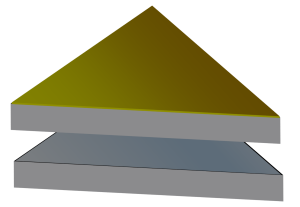Using a single, straight, knife-cut you wish to divide this cake into two pieces of equal volume. One solution might be to bisect it through the middle (as shown left). The problem with this strategy, however, is that the top of the cake is covered in delicious icing. Dividing the cake in this manner would result in one piece getting all the icing, and one piece getting none; this is not fair. We’re going to restrict ourselves into making cuts perpendicular to the top of the cake only.
 One way to do this is to bisect one of the corners and continue cutting across to the opposite side, where the cut exits normal to the edge. This clearly cuts the cake into two (equal) and opposite (chiral) slabs of cake. Well done! If the length of the side of the cake is one unit, then the length of the cut is √3/2 ≈ 0.8660 units. The question is, can you do better? Can you do it with a shorter cut?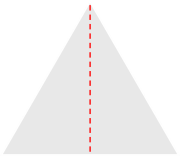What is the shortest straight line that will bisect an equilateral triangle?

The pieces don’t have to be the same shape, as long as they are the same area.

### Generic Cut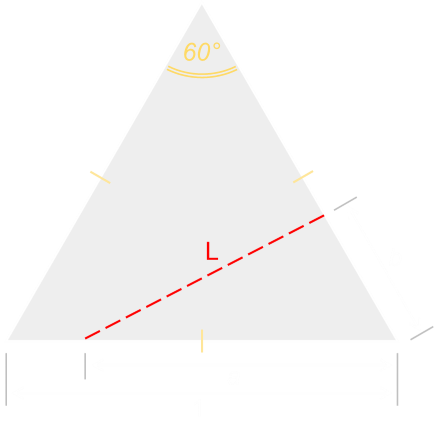We've already examined the case where the cut starts from a corner and goes to an edge (above). Now let's look at the generic case of a cut starting from an arbitrary location along one side, and ending somewhere on another edge (with rotations and reflections, this will cover all possible cases for a straight cut). This is shown on the left. We'll start the cut a units in from an arbitrary corner and it will end b units on an adjacent edge from the same corner. The length of the cut we'll define as L.Because we're going to assume the cake is of unifrom consistency in the vertical plane, to get equal volumes of cake, we just need to find a way to bisect the triangular plan of the cake.
 This cut will result in a triangular shaped piece, and a quadrilateral shaped piece. We need to find the relationship which results in both of these shapes having the same area.### AreaThe area of the top of the full cake is easy to calculate:When we cut the cake into two pieces, the area of each of these two pieces will be half the area of the cake. We can derive another formula for the smaller triangle based on the variables a and b.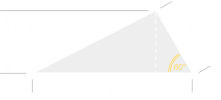Now let's look at the length of the cut L. For this we can use the cosine rule for triangles.Substituting in for b, we can get a formula for the length L, in terms of a:If we differtiate this, and set this to zero, we can find the turning point:The real positive roots for this equation give the results:This gives us the interesting result that a, b, and L are all the same value. We could probably have guessed at this result from symmetery, and what this means is the solution to the cake problem is to cut off a smaller equilateral triangle piece from the corner of the cake.

### ResultThe length of the cut, L, to make this triangle is 1/√2 ≈ 0.7071 (cf. 0.8660 when we bisect the corner). We've used calculus to find the shortest straight line cut.

# Arcs

What if we remove the restriction that the cut needs to be a straight line? If we are allowed to make our cut curved, is it possible to reduce the length of the cut? The answer is yes. Here is the proof, and the calculation:The first key to the proof is remembering that six equilateral triangles tesselate perfectly to form a regular hexagon. The second is the understanding that a circle is the most efficient container of area (This is the Isoperimetric Theorem): Among all planar shapes with the same area the circle has the shortest perimeter (or inversely: Among all planar shapes with the same perimeter the circle has the largest area).
 If we imagined placing six triangular cakes together we could then carve a circle out of the middle. From the Isoperimetric Theorem, we know the circumference of this circle will be the smallest to enclose his area inside it.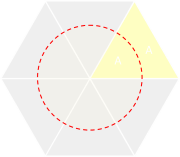What we need to do is determine the radius of the circle such that one sixth of the it's area is half of the area of the triangle.The circumference of a circle is 2πr, and the length of the arc cut is one sixth of this.### Result

Not only is this circular arc shorter than the straight line, we've shown that this arc is the shortest possible of any kind of curve.

### Even Shapes

Studying this is a little further, it's possible to show that, for shapes with an 'even' radial symmetery (such as squares, rectangles, circles …) the shortest curve to bisect a shape is a straight line that passes through the cetroid. For 'even' radial symmetery, any radius r, and any angle θ, (measured from the centroid) are the same, such that r(θ)=r(θ+π).

Any straight line passing through the centroid of an 'even' shape bisects that shape.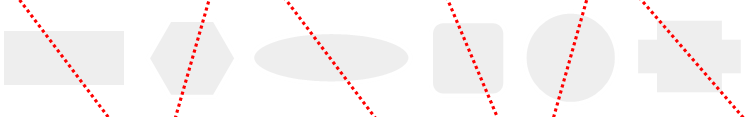This interesting result allows us to easily solve puzzles that, otherwise, seem quite complicated. Imagine that you have a tray of delicious brownies and want to divide it equally in half. Unfortunately, some evil person has cut out a smaller rectangle of brownies from the middle at some obscure angle. What is the easiest way to make the cut?
 The solution is to draw a straight line between the centroids of the two rectangles and make the cut along this line. From above, we know that a line passing through the centroid of an even shape bisects this shape. A line through both centroids bisects both shapes (both the cookie and the hole). Because of this, there will be the same amount of cookie on each side of the line.You can find a complete list of all the articles here.Click here to receive email alerts on new articles.